Measurement Error

Example Codes:

Henry Lu, Sylvia Li

Reliability Theory

Reliability refers to the consistency of a test or measurement. It shows the reproducibility of same test results in repeated trials. Better reliability implies higher precision of single measurement, as well as better tracking of changes in measurements in research or practical settings.

Reliability consists of absolute and relative consistency. Absolute consistency indicates the consistency of scores of individuals, whereas relative consistency concerns the consistency of the position or rank of individuals in the group relative to others.

Relative consistency is quantified through intraclass correlation coefficient (ICC). Absolute consistency is quantified by standard error of measurement (SEM) or variations such as minimum detectable difference (MDD) and standard error of prediction (SEP).

## Observed and true scores

The observed score is the number of points obtained on the test. Each observed score includes two parts, the true score, which is the mean of an infinite number of scores from the individual (ppt), and the error score, which is the difference between the observed score and true score.

## Reliability coefficient

For a group of measurements, the total variance (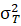) in the data is composed of true score variance (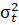) and error variance (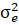), which can be expressed as: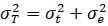The reliability coefficient is defined as: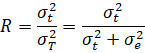The closer the reliability coefficient is to 1.0, the higher the reliability and the lower the.

Since the true score of each subject is not actually known,is used based on the between-subjects variability, i.e., the variance due to how subjects differ from each other. Thus, the formal definition of reliability coefficient becomes: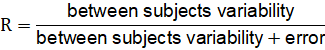The reliability coefficient could be quantified by various ICCs.

Intraclass Correlation Coefficient (ICC)

## Definition

ICC is a relative measure of reliability in that it is a ratio of variances derived from ANOVA, which represents the proportion of variance in a set of scores that is attributable to.

## Interpretation and properties

An ICC of 0.95 means that an estimated 95% of the observed score variance is due to the true score variance, and that an estimated 5% of the observed score variance is due to the error variance.

The magnitude of ICC depends on the between-subject variability, that ICC values are small when subjects differ little from each other, and vice versa.

ICC is unitless and theoretically varies between 0 and 1. ICC of 0 indicates no reliability, while ICC of 1 implies perfect reliability.

## Choices of ICC models

To calculate the ICC, the first step is to conduct a single-factor, within-subjects ANOVA. All subsequent equations are derived from the ANOVA table.

Shrout and Fleiss (1979) presented 6 forms of the ICC depends upon the experiment design:

 1-way random-effect model: Each subject is assumed to be assessed by a different set of raters, and raters are assumed to be randomly sampled from the population. Type of individual scores Shrout and Fleiss Convention Computational Formula Single score from each subject for each trail ICC (1,1)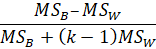Average of k scores from each subject ICC (1,k)2-way random-effect model: Each subject is assumed to be assessed by the same group of raters, and raters are assumed to be randomly sampled from the population. Type of individual scores Shrout and Fleiss Convention Computational Formula Single score from each subject for each trail ICC (2,1)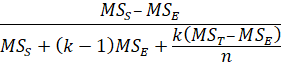Average of k scores from each subject ICC (2,k)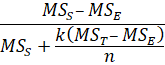2-way fixed-effect model: Each subject is assumed to be assessed by the same group of raters, and raters are only the raters of interest. Type of individual scores Shrout and Fleiss Convention Computational Formula Single score from each subject for each trail ICC (3,1)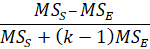Average of k scores from each subject ICC (3,k)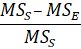# Standard Error of Measurement (SEM)

## Definition

The SEM is a determination of the amount of variation or spread in the measurement errors for a test, that it refers to the standard error in estimating observed scores from true scores. SEM has the same units as the measurement of interest and is usually used to define confidence intervals.

## Calculation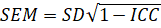where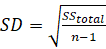is determined from the ANOVA.

## Confidence intervals of true scores and observed scores

The 95% confidence interval of observed score can be estimated as: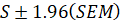Similarly, the 95% confidence interval of true score can be estimated as: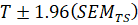where T is the estimated true score calculated as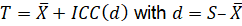and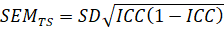# Minimum Detectable Difference (MDD)

## Definition

The MDD is the minimum statistically significant difference between measurements. For changes in the subject s scores which are at least greater than or equal to the MDD, 95% of them reflect real difference.

## Calculation

The SEM could be used to determine MDD as follows: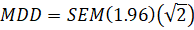# Standard Error of Prediction (SEP)

The SEP is used in defining the confidence intervals outside which one could be confident that a retest score reflects a real change in performance. The SEP and the 95% CI are computed as follows: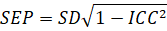The 95% CI is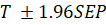, where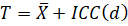is the estimated true score.

# Example code in SAS

data A;

call streaminit(123); /* set random number seed */

do i = 1 to 100;

r1 = rand("Normal",100,50); /* u ~ U(0,1) */

r2 = r1 + rand("Normal",10,10);

output;

end;

run;

data test_long;

set A;

array s(2) r:;

do judge = 1 to 2;

y = s(judge);

output;

end;

run;

%macro Icc_sas(ds, response, subject);

ods output OverallANOVA =all;

proc glm data=&ds;

class &subject;

model &response=&subject;

run;

data Icc(keep=sb sw n R R_low R_up);

retain sb sw n;

set all end=last;

if source='Model' then sb=ms;

if source='Error' then do;sw=ms; n=df; end;

if last then do;

R=round((sb-sw)/(sb+sw), 0.01);

vR1=((1-R)**2)/2;

vR2=(((1+R)**2)/n +((1-R)*(1+3*R)+4*(R**2))/(n-1));

VR=VR1*VR2;

L=(0.5*log((1+R)/(1-R)))-(1.96*sqrt(VR))/((1+R)*(1-R));

U=(0.5*log((1+R)/(1-R)))+(1.96*sqrt(VR))/((1+R)*(1-R));

R_Low=(exp(2*L)-1)/(exp(2*L)+1);

R_Up=(exp(2*U)-1)/(exp(2*U)+1);

output;

end;

run;

proc print data=icc noobs split='*';

var r r_low r_up;

label r='ICC*' r_low='Lower bound*' r_up='Upper bound*';

title 'Reliability test: ICC and its confidence limits';

run;

%mend;

%Icc_sas(test_long, response = y, subject = judge);

proc means data=test_long std;

var y;

run;

data;

icc = 0.44;

SD = 47.9575252;

SEM = SD * sqrt(1-icc);

SEM_TS = SD * sqrt(icc*(1-icc));

MD = SEM * 1.96 * sqrt(2);

MD_TS = SEM_TS * 1.96 * sqrt(2);

SEP = SD * sqrt(1-icc);

run;

# Example code in R

library(psych)

library(tidyverse)

# Simulate data.

# For the input data, you need a data frame that has 2 coloumns and n rows.

# This data can be any data - but repeat reading/measure from the same reader per row

set.seed(1)

n <- 100

r1 <- rnorm(n, mean = 100, sd = 50)

r2 <- r1 + rnorm(n, mean = 10, sd = 10)

data <- tibble(

)

# SEM and MDD function.

## For SEM, you need either ICC(2,1) or ICC(3,1).

get_sem_mdd <- function(data, icc_type){

# Calculate SEM - equation (8).

## Get SD first.

SD <- data %>%

pivot_longer(everything()) %>%

pluck('value') %>%

sd()

## Get ICC.

data_icc <- ICC(data)\$results

icc <- data_icc %>%

filter(

type %in% icc_type

) %>%

pluck('est')

## Calculate SEM

SEM <- SD * sqrt(1-icc)

## SEM_TS equation (11)

SEM_TS <- SD * sqrt(icc*(1-icc))

## MD equation (12)

MD <- SEM * qnorm(0.975) * sqrt(2)

MD_TS <- SEM_TS * qnorm(0.975) * sqrt(2)

## SEP equation (15)

SEP <- SD * sqrt(1-icc)

result <- tibble(

SD,

SEM,

SEM_TS,

MD,

MD_TS,

SEP

)

return(result)

}

get_sem_mdd(data, 'ICC2')

# References

1.     Harvill, L.M. (1991). Standard Error of Measurement. Educational Measurement: Issues and Practice, 10, 33-41.

2.     Weir J. P. (2005). Quantifying test-retest reliability using the intraclass correlation coefficient and the SEM. Journal of strength and conditioning research19(1), 231 240. https://doi.org/10.1519/15184.1

3.     Hopkins W. G. (2000). Measures of reliability in sports medicine and science. Sports medicine (Auckland, N.Z.)30(1), 1 15. https://doi.org/10.2165/00007256-200030010-00001

4.     Duquesne, S., Alalouni, U., Gr ff, T., Frische, T., Pieper, S., Egerer, S., Gergs, R., & Wogram, J. (2020). Better define beta-optimizing MDD (minimum detectable difference) when interpreting treatment-related effects of pesticides in semi-field and field studies. Environmental science and pollution research international27(8), 8814 8821. https://doi.org/10.1007/s11356-020-07761-0